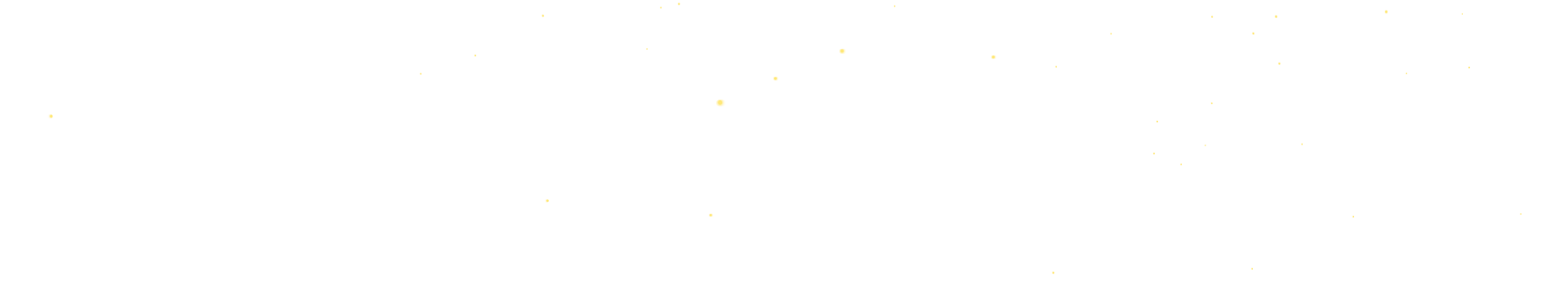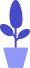# Python turtle module example: draw Peppa Pig (middle)

Estimated reading: 2 minutes 58 views

Following the previous tutorial, we continued to draw Peppa Pig.

## cheek() function

Next, we define the cheek() function, which draws a red circle to represent the blush. The cheek() function code is shown below.

``````def cheek():
penup()
goto(80,10)
color((255,155,192))
pendown()
begin_fill()
circle(30)
end_fill()``````

Call this function to see its drawing effect, as shown in Figure 1.figure 1

## mouth() function

Next we define the mouth() function, which is to draw a red arc to represent the mouth. The code for the mouth() function is shown below.

``````def mouth():
penup()
goto(-20,30)
color(239,69,19)
pendown()
circle(35,120)``````

Call this function to see the drawing effect, as shown in Figure 2.figure 2

## body() function

Next, we define the body() function, which is used to draw the body. The code for the body() function is shown below.

``````def body():
color("red",(255,99,71))
#The curve on the left side of the body
penup()
goto(-32,-8)
pendown()
begin_fill()
circle(100,10)
circle(300,30)
#body bottom
forward(230)
#The curve on the right side of the body
circle(300,30)
circle(100,3)
color((255,155,192),(255,100,100))
#Draw the chin on the face to avoid covering it when filling
circle(-80,63)
circle(-150,24)
end_fill()``````

Call this function to see the drawing effect, as shown in Figure 3.image 3

## hands() function

Next, we define the hands() function to draw hands. The code for the hands() function is shown below.

``````def hands():
color((255,155,192))
# middle finger of left hand
penup()
goto(-56,-45)
pendown()
circle(300,15)
# Represent the other two fingers of the left hand through an arc
penup()
forward(15)
pendown()
circle(-20,90)
# middle finger of right hand
penup()
forward(30)
forward(237)
pendown()